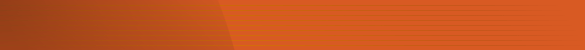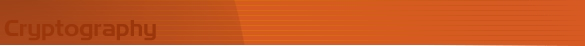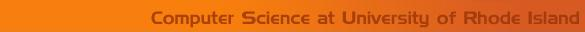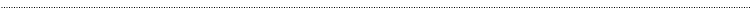Home Page Introduction Classical Ciphers Enigma Machine DES Key Agreement PGP R.S.A. Number Theory Other LinksPublickey CryptographyDiffie- Hellman Key Agreement Protocol Diffie-Hellman Key Agreement protocol  can be used to exchange keys for a symmetric cryptosystem over an insecure channel Setup p is a large prime number called the public prime s is a number in the range of 2 to p-1, called the public base Alice chooses a number a at random in the range of 2 to p-2, then calculates A = sa mod p and sends it to Bob Bob chooses a number b at random in the range of 2 to p-2, then calculates B = sb mod p and sends it to Alice   Key Retrieval Alice calculates k = Ba mod p = (sb)a mod p = sba mod p Bob calculates k = Ab mod p = (sa)b mod p = sab mod p Now both Alice and Bob have the same key k and they can use it in a symmetric cryptosystem like DES. Since Alice knows a and B and Bob knows b and A,  both of them can calculate sab. But someone with the knowledge of p, s, A, B will not be able to calculate the key k The Diffie- Hellman Problem Find sab mod p from sa and sb in a time-efficient manner. It is obvious that for an eavesdropper, Eve, to find the key k, she has to find a and b given sa = A mod p and  Sb = B mod p . There is no such efficient algorithm to solve for a and b. The Diffie- Hellman problem is still an open question without an efficient solution. You might want to try to crack it, rest assured you and your algorithm will be more famous than any of the existing algorithms related to cryptography. Good luck!   Example Public prime p = 79 Public base   s = 56 Alice chooses a= 35 Alice calculates A  = sa mod p = (56)35 mod 79 =  15366711764103772265936719131269189389773889471090594389426176 mod 79 = 24 Bob chooses b= 12 Bob calculates B  = sb mod p  =  (56)12 mod 79 = 951166013805414055936 mod 79 = 1 Alice calculates key  k =  Ba mod p = (1)35 mod 79 = 1 mod 79 = 1 Bob calculates key k = Ab mod p  =  (24)12 mod 79 = 36520347436056576  mod 79 = 1